Test Description

## 10 Questions MCQ Test Science Olympiad Class 9 | Olympiad Test: Motion- 2

Olympiad Test: Motion- 2 for Class 9 2022 is part of Science Olympiad Class 9 preparation. The Olympiad Test: Motion- 2 questions and answers have been prepared according to the Class 9 exam syllabus.The Olympiad Test: Motion- 2 MCQs are made for Class 9 2022 Exam. Find important definitions, questions, notes, meanings, examples, exercises, MCQs and online tests for Olympiad Test: Motion- 2 below.
 1 Crore+ students have signed up on EduRev. Have you?
Olympiad Test: Motion- 2 - Question 1

### If a body moves 6 m towards South and then turns towards East and moves 8 m, then find the displacement of the body

Detailed Solution for Olympiad Test: Motion- 2 - Question 1

Applying Pythagoras

theorem,

OQ = √(OP2+PQ 2)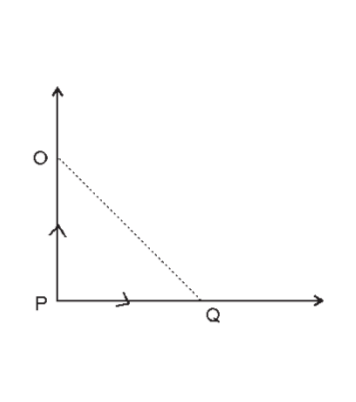=√(36+64)=10m

Since,OQ is the actual displacement.

Olympiad Test: Motion- 2 - Question 2

### In the speed-time graph for a moving object shown here, the part which indicates uniformdeceleration of the object is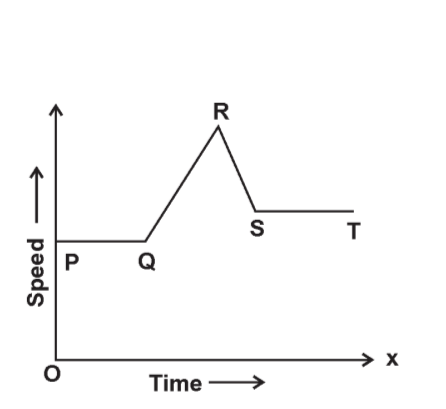Detailed Solution for Olympiad Test: Motion- 2 - Question 2

As we move along the graph, PQ and ST show uniform acceleration. QR shows acceleration at an increasing rate. RS shows deceleration i.e.,speed is decreasing.

Olympiad Test: Motion- 2 - Question 3

### An artificial satellite is moving in a circular orbit of radius 32,000 km. If it takes 30 hours to complete one revolution around the earth, then find the velocity of the satellite

Detailed Solution for Olympiad Test: Motion- 2 - Question 3

R = 32000 km, t = 30 hours

Circumference of the orbit = 2πR

=2*(22/7)*32000

=201142.86km

Velocity=d/t=2πR/t=6704 km/h

Olympiad Test: Motion- 2 - Question 4

A man travels a distance of 2 m towards East, 6 m towards South and finally 6m towards the East. The resultant displacement is

Detailed Solution for Olympiad Test: Motion- 2 - Question 4

A man starts from O and reaches Q. Shortest distance is OQ.

Apply Pythagoras theorem to

∆ORQ.

(RQ = PM) and (PR = MQ)()

OQ2= OR2+ RQ2

OQ=√{(2+6)2+62}

OQ=10m

Olympiad Test: Motion- 2 - Question 5

The area under a speed–time graph represents a physical quantity which has the unit

Detailed Solution for Olympiad Test: Motion- 2 - Question 5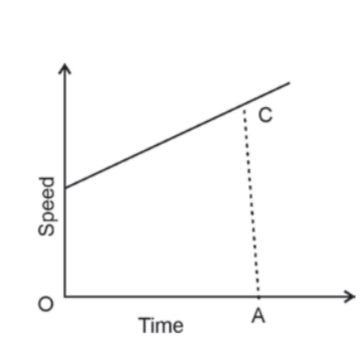Area of trapezium ={(OB+AC)*OA}/2

= Distance travelled in A seconds, whose unit is m. Initial speed = OB, then accelerating from B to C in time A.

Olympiad Test: Motion- 2 - Question 6

The motion in which a body has a constant speed but not constant velocity is called

Detailed Solution for Olympiad Test: Motion- 2 - Question 6

As the direction keeps on changing continuously in a circular motion, the speed remains constant but velocity keeps on changing all the time.

Olympiad Test: Motion- 2 - Question 7

What can you say about the motion of the body if its speed–time graph is a straight line parallel to the time axis ?

Detailed Solution for Olympiad Test: Motion- 2 - Question 7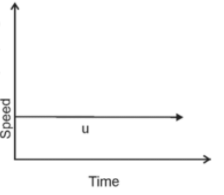In the case of the speed–time graph, having a constant line parallel to the time (x) axis, we see that speed is fixed (constant) but time keeps on moving in this graph as shown,Speed = u (throughout).

Olympiad Test: Motion- 2 - Question 8

The figure shows distance–time graphs of two cars A and B running at different speeds. Which car is running with a greater speed in comparison to the other car?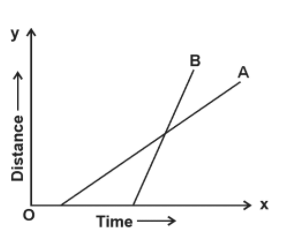Detailed Solution for Olympiad Test: Motion- 2 - Question 8

The line graph for car B makes a larger angle with time –axis. Its slope is larger than the slope of the line for car A. And the slope of distance–time graph shows speed. Thus car B has greater speed than car A.

Olympiad Test: Motion- 2 - Question 9

A car accelerates from 15 km/h to 60 km/h in 300 seconds. Find the distance travelled by the car during this time

Detailed Solution for Olympiad Test: Motion- 2 - Question 9

u = 15 km/h, v = 60 km/h, t = 300 sec =1/12 h

a=(v-u)/t =(60-15)/(1/12)=540 km/h2

Distance travelled by the car is given by

S=ut+(1/2)at2

=15*(1/12) + (1/2)*540*(1/2)

=5 km

Olympiad Test: Motion- 2 - Question 10

A motorcycle is being driven at a speed of 20 m/s when brakes are applied to bring it to rest in five seconds. The deceleration produced in this case will be

Detailed Solution for Olympiad Test: Motion- 2 - Question 10

v = 0 u = 20 m/s, t = 5 s; a

=(v-u)/t =(0-20)/5= – 4 m/s2